# Custimize the pulse-level simulation¶

Author: Boxi Li ([email protected])

In this note, we demonstrate examples of customizing the pulse-level simulator in qutip-qip.The notebook is divided into three parts:

1. Customizing the Hamiltonian model
2. Customizing the compiler
3. Customizing the noise
In :
# imports
import numpy as np

from qutip import sigmax, sigmay, sigmaz, tensor, fidelity
from qutip import basis

from qutip_qip.pulse import Pulse
from qutip_qip.device import ModelProcessor, Model
from qutip_qip.circuit import QubitCircuit
from qutip_qip.compiler import GateCompiler, Instruction, SpinChainCompiler
from qutip_qip.noise import Noise

import matplotlib.pyplot as plt


## Customizing the Hamiltonian model¶

We start from the customization of the Hamiltonian model, which is represented by a class instance of Model.A minimal requirement for a processor model is specifying the hardware parameters and the control Hamiltonians of the physical system. The former will be provided to the compiler for computing the pulse strength. The latter will be accessed by the Processor class via the method get_control.

In :
class MyModel(Model):
def __init__(self, num_qubits, dims=None, h_x=1., h_z=1., g=0.1, t1=None, t2=None):
super().__init__(num_qubits, dims=dims)
self.params = {
"sz": [h_z] * num_qubits,
"sx": [h_x] * num_qubits,
"g": [g] * num_qubits,
"t1": t1, #  Will be accessed by the noise module.
"t2": t2,
}
# Control Hamiltonians
_two_qubit_operator = tensor([sigmax(), sigmax()]) + tensor([sigmay(), sigmay()])
self.controls = {}
self.controls.update(
{f"sx{n}": (2 * np.pi * sigmax(), n) for n in range(num_qubits)})
self.controls.update(
{f"sz{n}": (2 * np.pi * sigmaz(), n) for n in range(num_qubits)}),
self.controls.update(
{f"g{n}": (2 * np.pi * _two_qubit_operator, [n, n+1]) for n in range(num_qubits - 1)}),

def get_control(self, label):
"""
The mandatory method. It Returns a pair of Qobj and int representing the control Hamiltonian and the target qubit.
"""
return self.controls[label]

def get_control_labels(self):
"""
It returns all the labels of availble controls.
"""
return self.controls.keys()

def get_control_latex(self):
"""
The function returns a list of dictionaries, corresponding to the latex representation of each control.
This is used in the plotting. Controls in each dictionary will be plotted in a different colour.
See examples later in this notebook.
"""
return ([
{f"sx{n}": r"$\sigma_x^%d$" % n for n in range(num_qubits)},
{f"sy{n}": r"$\sigma_z^%d$" % n for n in range(num_qubits)},
{f"g{n}": r"$g_{%d}$" % (n) for n in range(num_qubits - 1)}])


This is a quantum system of $n$ qubits arranged in a chain (same as the spin chain model), where we have control over three Hamiltonian: $\sigma_x$, $\sigma_z$ on each qubit, and neighbouring-qubits interaction $\sigma_x\sigma_x+\sigma_y\sigma_y$:

$$H = \sum_{j=0}^{n-1} c_{1,j}(t) \cdot h_x^{j}\sigma_x^{j} + \sum_{j=0}^{n-1} c_{2,j}(t) \cdot h_z^{j}\sigma_z^{j} + \sum_{j=0}^{n-2} c_{3,j}(t)\cdot g^{j}(\sigma_x^{j}\sigma_x^{j+1}+\sigma_y^{j}\sigma_y^{j+1})$$

where $h_x$, $h_z$, $g$ are the hardware parameters and $c_{i,j}(t)$ are the time-dependent control pulse coefficients. This Hamiltonian is the same as the one for the linear spin chain model in QuTiP. In general, the hardware parameters will not be identical for each qubit, but here, for simplicity, we represent them by three numbers: $h_x$, $h_z$ and $g$.

To simulate a custom quantum device, we provide the model to ModelProcessor, which is used for simulators based on a concrete physics model (in contrast to optimal control for arbitrary Hamiltonians). In this way, we inherit the necessary methods from ModelProcessor used in the simulation.

In :
num_qubits = 2
processor = ModelProcessor(model=MyModel(num_qubits, h_x=1.0, h_z=1.0, g=0.1))


In set_up_ops, we define the control Hamiltonians of the quantum system and use them to initialize the control pulses. We can get the map between the pulse labels and the pulse location with

In :
processor.get_control_labels()

Out:
dict_keys(['sx0', 'sx1', 'sz0', 'sz1', 'g0'])

Or access a control Hamiltonian by its label:

In :
sx0 = processor.get_control("sx0")
sx0

Out:
(Quantum object: dims = [, ], shape = (2, 2), type = oper, isherm = True
Qobj data =
[[0.         6.28318531]
[6.28318531 0.        ]],
0)

In qutip-qip 0.1 version, the model is directly defined in the Processor. This can still be achieved in version 0.2 as shown in the following cell. This is equivalent to the definition above. An instance of Model is automatically created and saved in the processor.

In :
class MyProcessor(ModelProcessor):
"""
Custom processor built using ModelProcessor as the base class.
This custom processor will inherit all the methods of the base class
such as setting up of the T1 and T2 decoherence rates in the simulations.

Args:
num_qubits (int): Number of qubits in the processor.
t1, t2 (float or list): The T1 and T2 decoherence rates for the
"""
def __init__(self, num_qubits, h_x, h_z, g, t1=None, t2=None):
super(MyProcessor, self).__init__(num_qubits, t1=t1, t2=t2)  # call the parent class initializer
self.pulse_mode = "discrete"  # The control pulse is discrete or continous.
self.model.params.update({"sz": [h_z] * num_qubits,  # can also be different for each qubit
"sx": [h_x] * num_qubits,
"g": [g] * num_qubits})
self.model.dims =  * num_qubits  # The dimension of each controllable quantum system, here num_qubits qubits.
self.num_qubits = num_qubits
self.set_up_ops()  # set up the available Hamiltonians

def set_up_ops(self):
"""
Sets up the control operators.
"""
for m in range(self.num_qubits):
# sigmax pulse on m-th qubit with the corresponding pulse
self.add_control(2 * np.pi * sigmax(), m, label="sx" + str(m))
# sz
for m in range(self.num_qubits):
self.add_control(2 * np.pi * sigmaz(), m, label="sz" + str(m))
# interaction operator
operator = tensor([sigmax(), sigmax()]) + tensor([sigmay(), sigmay()])
for m in range(self.num_qubits - 1):
self.add_control(2 * np.pi * operator, [m, m+1], label="g" + str(m))


### Load and compile the circuit¶

We first define a quantum circuit. Here we use a two-qubit circuit with two X gates. Acting on a $|00\rangle$ state, this results in a $|11\rangle$ state.

In :
circuit = QubitCircuit(num_qubits)
circuit

Out:For circuit plotting, see this notebook.

To convert a quantum circuit into the Hamiltonian model, we need a compiler. The custom definition of a compiler will be discussed in details in the next section. Because we used the Hamiltonian model of the spin chain, we here simply "borrow" the compiler of the spin chain model.

In :
processor = ModelProcessor(model=MyModel(num_qubits, h_x=1.0, h_z=1.0, g=0.1))
processor.native_gates = ["ISWAP", "RX", "RZ"]

# processor.num_qubits, processor.params access directly the information in the model.
compiler = SpinChainCompiler(processor.num_qubits, processor.params)

result = processor.run_state(init_state = basis([2,2], [0,0]))
result.states[-1]

Out:
Quantum object: dims = [[2, 2], [1, 1]], shape = (4, 1), type = ket\begin{equation*}\left(\begin{array}{*{11}c}3.450\times10^{-07}\\1.624\times10^{-08}j\\1.624\times10^{-08}j\\-1.000\\\end{array}\right)\end{equation*}

The compiled pulse coefficients are now saved in the processor and can be accessed by:

In :
sx1_pulse = processor.find_pulse("sx1")
print(sx1_pulse.coeff)
print(sx1_pulse.tlist)

[1.]
[0.   0.25]


This is a rectangular pulse that starts from time 0 and ends at time 0.25.

#### Note¶

For discrete pulse, the time sequence is one element shorter than the pulse coefficient because we need to specify the start and the end of the pulse. If two sequences are of the same length, the last element of coeff will be neglected. Later, we will see continuous pulse where coeff and tlist have the same length.

To give an intuitive illustration of the control pulses, we give each pulse a latex label by defining a method get_operators_labels and then plot the compiled pulses.

In :
processor.plot_pulses()
plt.show()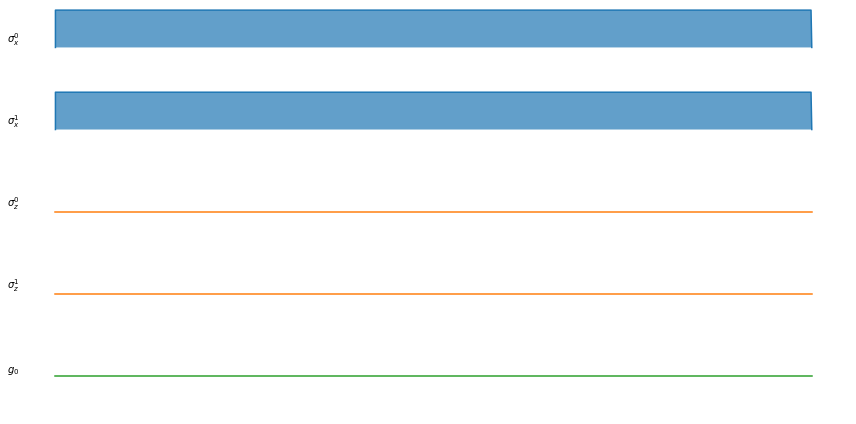## Customizing the compiler¶

How the quantum gates are implemented on hardware varies on different quantum systems. Even on the same physical platform, different implementation will yield different performance. The simplest way of implementation is to define a rectangular pulse like the one above. However, in reality, the control signal will have a continuous shape. In the following, we show how to customize the compiler with a gaussian pulse.

A typical gate compiler function looks like the one in the following cell, with the form XX_compiler(self, gate, args). It takes two arguments, gate and args: gate is the quantum gate to be compiled and args is a dictionary for additional parameters, for instance, parameters we defined in Processor.params.

For each gate, the function returns the input gate, the time sequence and the pulse coefficients in an Instruction object.

Below is an example of a rectangular pulse.

In :
def rz_compiler(gate, args):
"""
Compiles the RZ gate to an instruction for a pulse.

Args:
gate (qutip_qip.circuit.Gate): A qutip Gate object.
args:(dict): A dictionary for compilation arguments e.g. hardware parameters.

Returns:
Instruction (qutip_qip.compiler.instruction.Instruction): An instruction to implement
a gate containing the control
pulses.
"""
tlist = np.array([1.])
coeff = np.array([0., 0.25])
# instruction is an object that includes the pulse coefficient and time sequence
pulse_info = [("sz0", coeff)]
return [Instruction(gate, tlist, pulse_info)]


Now we replace it with a continuous pulse. In particular, we define a single_qubit_compiler method. For RX and RY gate, it reads the maximal driving strength from the parameters and computes the corresponding time sequence and pulse amplitude.

For simplicity, we make use of the generate_pulse_shape method, which is defined in the parent class GateCompiler and is available in all subclasses.

In :
class MyCompiler(GateCompiler):  # compiler class
def __init__(self, num_qubits, params):
super(MyCompiler, self).__init__(num_qubits, params=params)
# pass our compiler function as a compiler for X gate.
self.gate_compiler["X"] = self.single_qubit_compiler
self.gate_compiler["Y"] = self.single_qubit_compiler
self.args.update({"params": params})

def single_qubit_compiler(self, gate, args):
"""
Compiler for the X and Y gate.
"""
targets = gate.targets
if gate.name == "Z":
pulse_prefix = "sz"
pulse_strength = args["params"]["sz"][targets]
elif gate.name == "X":
pulse_prefix = "sx"
pulse_strength = args["params"]["sx"][targets]
coeff, tlist= self.generate_pulse_shape(
"hann",  # Scipy Hann window
100,  # 100 sampling point
maximum=pulse_strength,
area= (np.pi/2) / (2*np.pi),  # 1/2 becuase we use sigmax as the operator instead of sigmax/2
)
sign = np.sign(pulse_strength)
pulse_info = [(pulse_prefix + str(targets), coeff)]
return [Instruction(gate, tlist, pulse_info)]


The attribute pulse_mode can be set to "discrete" or "continuous". For continuous pulse, QuTiP solvers will use cubic spline interpolation. To minimize the boundary effect, it is recommended that the continuous pulse goes smoothly to 0 at the beginning and the end. We can see the advantage of the scheduling when we introduce a T1 decoherence and compare the fidelity of the final state.

In :
gauss_compiler = MyCompiler(processor.num_qubits, processor.params)
processor = ModelProcessor(model=MyModel(num_qubits, h_x=1.0, h_z=1.0, g=0.1, t1=300))
processor.pulse_mode = "continuous"
tlist, coeff = processor.load_circuit(circuit, compiler=gauss_compiler, schedule_mode = False);
print
result = processor.run_state(init_state = basis([2,2], [0,0]))
print("fidelity without scheduling:", fidelity(result.states[-1], basis([2,2],[1,1])))

fidelity without scheduling: 0.9982136886930341

In :
processor.plot_pulses(use_control_latex=False)
plt.show()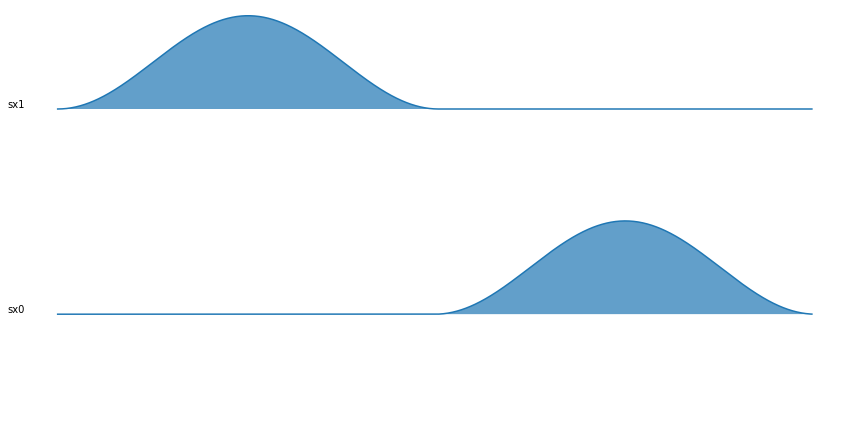We can also turn on the scheduler to schedule the circuit for a shorter execution time.

In :
processor = ModelProcessor(model=MyModel(num_qubits, h_x=1.0, h_z=1.0, g=0.1, t1=300))
tlist, coeffs = processor.load_circuit(circuit, schedule_mode = "ASAP", compiler=gauss_compiler);
processor.pulse_mode = "continuous"
result = processor.run_state(init_state = basis([2,2], [0,0]))
print("fidelity with scheduling:", fidelity(result.states[-1], basis([2,2],[1,1])))

fidelity with scheduling: 0.9990450021201522

In :
processor.plot_pulses(use_control_latex=False)
plt.show()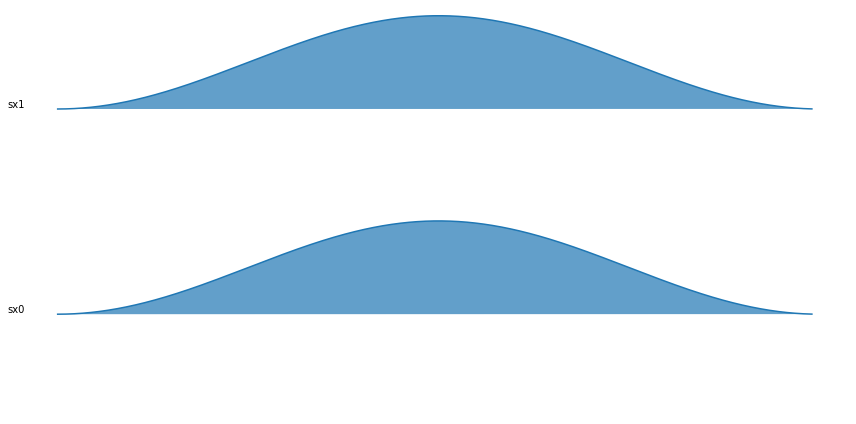The number of predefined gates in qutip is limited, hence you are encouraged to define your own gate. Here we show how to define a parametrized gate. First, you will need to define a gate function:

In :
def mygate(theta = None):
# We just call the Molmer Sorensen gate as an example.
# If you do not want to run the circuit at the gate matrix level (circuit.run), no need for this function,
# otherwise you will need to define this python function that returns the Qobj of the gate.)
from qutip_qip.operations import molmer_sorensen
return molmer_sorensen(theta, 2, targets=[0, 1])
circuit = QubitCircuit(2)
circuit.user_gates = {"MYGATE": mygate}  # no need for this if you don't use circuit.run
circuit
# You may see a warning because MYGATE is not found in defined LaTeX gate names, just ignore it.

E:\Miniconda3\envs\notebook\lib\site-packages\qutip_qip\operations\gates.py:463: UserWarning: Unknown gate MYGATE
warnings.warn("Unknown gate %s" % name)

Out: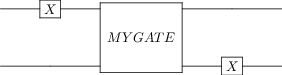In :
circuit.run(basis([2,2],[0,0]))

Out:
Quantum object: dims = [[2, 2], [1, 1]], shape = (4, 1), type = ket\begin{equation*}\left(\begin{array}{*{11}c}-0.707j\\0.0\\0.0\\-0.707\\\end{array}\right)\end{equation*}

The next step is to define a compiler function for it. Since compiling a real MS gate is rather complicated, we here just use a dummy triangular pulse. The goal is to show how to get access to the parameter theta during the compiling.

In :
def mygate_compiler(gate, args):
targets = gate.targets  # target qubit
parameters = args["params"]

theta = gate.arg_value
coeff1 = np.concatenate([np.linspace(0,10,50), np.linspace(10,0,50), ]) / 50
coeff2 = np.concatenate([np.linspace(0,10,50), np.linspace(10,0,50), ]) / 50
pulse_info = [("sx" + str(targets), theta * coeff1),
("sx" + str(targets), theta * coeff2)
]  #  save the information in a tuple (pulse_name, coeff)
tlist = np.linspace(0, 1, len(coeff1))
return [Instruction(gate, tlist, pulse_info)]

In :
gauss_compiler = MyCompiler(processor.num_qubits, processor.params)
processor = ModelProcessor(model=MyModel(num_qubits, h_x=1.0, h_z=1.0, g=0.1, t1=300))
gauss_compiler.gate_compiler["MYGATE"] = mygate_compiler
processor.pulse_mode = "continuous"
processor.plot_pulses()
plt.show()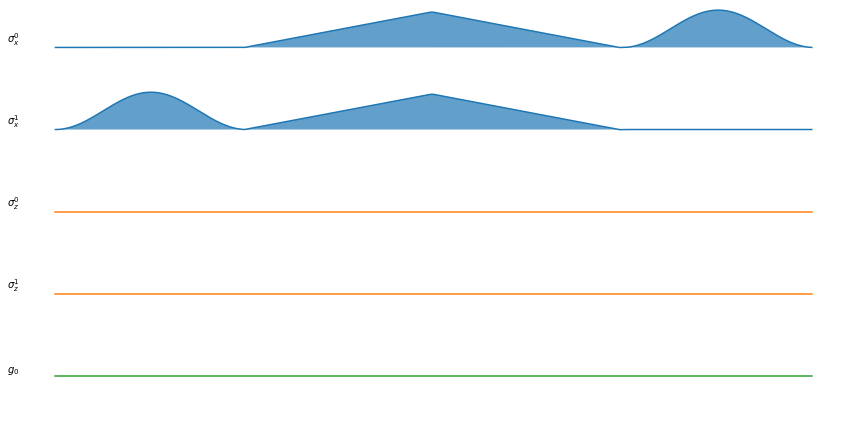## Customizing the noise¶

Apart from pre-defined noise such as T1, T2 noise and random noise in the control pulse amplitude (see this notebook, one can also define custom noise. Here we will see two examples of customizing noise, one systematic (pulse-independent) noise and one pulse-dependent noise.

To understand how noise is processed, we briefly introduced the data structure of the simulation framework. The control elements are stored as a list of Pulse objects in the Processor. In each Pulse contains the idea pulse, the control noise part and the decoherence part. For systematic noise, it is saved under the Pulse representation labelled "system", which represents the intrinsic dynamics of the quantum system. For pulse-dependent noise, we will add them to their corresponding control Pulse.

The definition of noise is realized by a subclass of UserNoise, including two methods:

• the initialization method containing the property of the noise, such as frequency or amplitude.
• the method get_noisy_dynamics that takes all the control pulse pulses, a dummy Pulse object representing systematic noise and the dimension of the system (here two qubits [2,2]).
In :
class Extral_decay(Noise):
def __init__(self, arg):
self.arg = arg
pass

def get_noisy_dynamics(self, dims, pulses, systematic_noise):
pass


### Systematic noise¶

We first show an example of systematic noise. Here, we introduce a ZZ crosstalk noise between neighbouring qubits with a constant strength. It is done in three steps:

• Define the noise class.
• Initialize the noise object with given coupling strength.
• Define the Processor as usual and add the noise to the processor.

In the following example, we check the fidelity of the same circuit of two X gates, but now with this additional noise.

In :
circuit = QubitCircuit(2)

In :
from qutip import sigmaz
from qutip_qip.operations import expand_operator
from qutip_qip.pulse import Pulse
from qutip_qip.noise import Noise

class ZZ_crosstalk(Noise):
def __init__(self, strength):
self.strength = strength

def get_noisy_dynamics(self, dims, pulses, systematic_noise):
zz_operator = tensor([sigmaz(), sigmaz()])
for i in range(len(dims)-1):
self.strength * zz_operator, targets=[i,i+1], tlist=None, coeff=True)  # constant, always 1

crosstalk_noise = ZZ_crosstalk(strength=1.0)

In :
processor = ModelProcessor(model=MyModel(num_qubits, h_x=1.0, h_z=1.0, g=0.1))
gauss_compiler = MyCompiler(processor.num_qubits, processor.params)

result = processor.run_state(init_state = basis([2,2], [0,0]))
print("Final fidelity with ZZ crosstalk:", fidelity(result.states[-1],basis([2,2],[1,1])))

Final fidelity with ZZ crosstalk: 0.9899565784438967


### Pulse dependent noise¶

In this second example, we demonstrate how to add an additional amplitude damping channel on the qubits. The amplitude of this decay is linearly dependent on the control pulse "sx", i.e. whenever the pulse "sx" is turned on, the decoherence is also turned on. The corresponding annihilation operator has a coefficient proportional to the control pulse amplitude. This noise can be added on top of the default T1, T2 noise.

In :
from qutip_qip.pulse import Pulse
class Extral_decay(Noise):
def __init__(self, ratio):
self.ratio = ratio

def get_noisy_dynamics(self, dims, pulses, systematic_noise):
from qutip import destroy
op = destroy(2)
for pulse in pulses:   # iterate for all pulses
if "sx" in pulse.label and pulse.coeff is not None:   # if it is a sigma-x pulse and is not empty

extral_decay = Extral_decay(0.3)

In :
processor = ModelProcessor(model=MyModel(num_qubits, h_x=1.0, h_z=1.0, g=0.1))
gauss_compiler = MyCompiler(processor.num_qubits, processor.params)

result = processor.run_state(init_state = basis([2,2], [0,0]))
print("Final fidelity with pulse dependent decoherence:", fidelity(result.states[-1],basis([2,2],[1,1])))

Final fidelity with pulse dependent decoherence: 0.9939250019133458

In :
import qutip_qip
print(qutip_qip.version.version)
from qutip.ipynbtools import version_table
version_table()

0.2.0

Out:
SoftwareVersion
QuTiP4.6.3
Numpy1.22.2
SciPy1.8.0
matplotlib3.5.1
Cython0.29.27
Number of CPUs12
BLAS InfoOPENBLAS
IPython8.0.1
Python3.9.0 | packaged by conda-forge | (default, Nov 26 2020, 07:53:15) [MSC v.1916 64 bit (AMD64)]
OSnt [win32]
Sat Feb 12 23:26:05 2022 W. Europe Standard Time